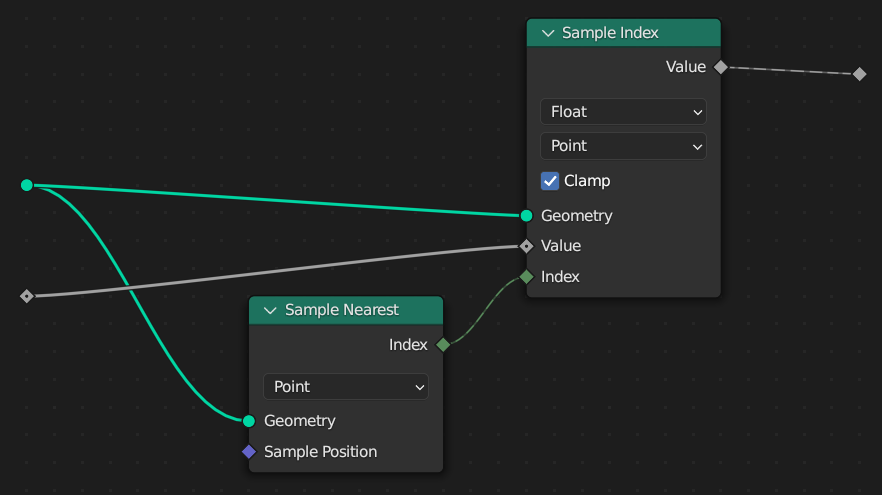# Sample Nearest Node

The Sample Nearest node retrieves the index of the geometry element in its input geometry that is closest to the input position.

This node is similar to the Geometry Proximity(ジオメトリ近接)ノード, but it outputs the index of the closest element instead of its distance from the current location.

Tip

If you want to find nearest to each point in same geometry, its better to use the Index of Nearest node.

## 入力

Geometry(ジオメトリ)

The geometry to sample.

This node only supports point cloud and mesh inputs.

Sample Position

The position to start from when finding the closest location on the target geometry. By default, this is the same as if the Position(位置)ノード was connected.

## Properties(プロパティ)

Domain(ドメイン)

The attribute domain to consider the distance from.

## 出力

Index(インデックス)

The index of the closest geometry element of the chosen domain.

## 例Combining this node with the Sample Index Node gives a setup that can retrieve the closest attribute value from another geometry. This is the same behavior as the Transfer Attribute node in versions of Blender before 3.4.